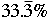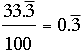SEARCH HOMEMath Central Quandaries & QueriesQuestion from Traci, a parent: Please convert 66 2/3% into a decimal and a fractionTraci,

I'm going to use 33 1/3% and let you work with 66 2/3%.

First I divide 3 into 1 to get 1/3 = 0.333 where the 3 is repeating. Hence 33 1/3% isBut "percent" means per one-hundred sois. As a fraction that's 1/3.

PennyMath Central is supported by the University of Regina and The Pacific Institute for the Mathematical Sciences.